# Medical tricorder Mark II

Gerber_Medical_Tricorder_MK2.zip (370074Bytes)
Medical_tricorder_main_code_1.zip (6092Bytes)
Connection_nipple.zip (98938Bytes)
Spectrophotometer_3-D.zip (611601Bytes)

Update March 15, 2015: My medical tricorder project is now a Hackaday Prize 2015 entry

1. Introduction

A medical tricorder is a handheld portable scanning device to be used by consumers to self-diagnose medical conditions within seconds and take basic vital measurements . The word “tricorder” is an abbreviation of the device’s full name, the “TRI-function reCORDER”, referring to the device’s primary functions; Sensing, Computing and Recording .

We will sense the 3 basic vital signs, which are body temperature, pulse rate and respiration rate. We will process these data using naive Bayes classifiers trained  in a supervised learning setting for medical diagnosis beside other tools.

2. Directly diagnosed diseases

Following diseases can be directly diagnosed, just comparing measured data to look-up-tables:

 Body temperature Heart rate Respiratory Rate Direct diagnoses Hypothermia Fever (Hyperthermia, Hyperpyrexia) Bradycardia Tachycardia Bradypnea Tachypnea

Temperature classification 

 Class Body core temperature Hypothermia < 35 °C Normal 36.5-37.5 °C Fever > 38.3 °C Hyperthermia > 40.0 °C Hyperpyrexia > 41.5 °C

Resting heart rate 

 Age Resting heart rate 0-1 month 70-190 bpm 1-11 months 80-160 bpm 1-2 years 80-130 bpm 3-4 years 80-120 bpm 5-6 years 75-115 bpm 7-9 years 70-110 bpm > 10 years 60-100 bpm (Well-trained athletes: 40-60 bpm)

Respiratory rate 

 Age Respiratory Rate 0-2 months 25-60 bpm 3-5 months 25-55 bpm 6-11 months 25-55 bpm 1 year 20-40 bpm 2-3 years 20-40 bpm 4-5 years 20-40 bpm 6-7 years 16-34 bpm 8-9 years 16-34 bpm 10-11 years 16-34 bpm 12-13 years 14-26 bpm 14-16 years 14-26 bpm ≥ 17 years 14-26 bpm

3. Naive Bayes classifier at a glance

There are many tutorials about the naive Bayes classifier out there, so I keep it short here.

Bayes' theorem: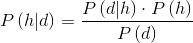h: Hypothesis
d: Data
P(h): Probability of hypothesis h before seeing any data d
P(d|h): Probability of the data if the hypothesis h is true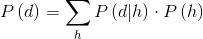: Data evidence
P(h|d): Probability of hypothesis h after having seen the data d

Generally we want the most probable hypothesis given training data. This is the maximum a posteriori hypothesisH: Hypothesis set or space

As the denominators P(d) are identical for all hypotheses, hMAP can be simplified:If our data d has several attributes, the naïve Bayes assumption can be used. Attributes a that describe data instances are conditionally independent given the classification hypothesis: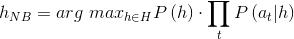4. Common cold/flu classifier

Every human depending on the age catches a cold 3-15 times a year. Taking the average 9 times a year and assuming a world population of 7·109, we have 63·109 common cold cases a year. Around 5·106 people will get the flu per year. Now we can compute:This means only one of approx. 12500 patients with common cold/flu like symptoms has actually flu! Rests of the data are taken from here. The probability-look-up table for supervised learning looks then as follows:

 Prob Flu Common cold P(h) 0.00008 0.99992 P(Fatigue|h) 0.8 0.225 P(Fever|h) 0.9 0.005 P(Chills|h) 0.9 0.1 P(Sore throat|h) 0.55 0.5 P(Cough|h) 0.9 0.4 P(Headache|h) 0.85 0.25 P(Muscle pain|h) 0.675 0.1 P(Sneezing|h) 0.25 0.9Let's do an example:Is it more likely that I suffer from common cold or flu?

Note: The probability that the event A is not occurring is given byAs 0.0004236973515 > 0.00000000868725, it is more likely that I suffer from a common cold.

We can already see, the numbers are quite small. Multiplying a lot of probabilities, which are between 0 and 1 by definition, can result in floating-point underflow. Since log⁡(x∙y)=log⁡(x)+log⁡(y), it is better to perform all computations by summing logs of probabilities rather than multiplying probabilities. The class with highest final un-normalized log probability score is still the most probable:An according sketch using the serial monitor and computer keyboard as interface would look like this:

```void setup() {
Serial.begin(9600);
}
void loop() {
flu_cold_classifier();
}
void diagnosis(boolean fatigue, boolean fever, boolean chills, boolean sore_throat,
boolean cough, boolean headache, boolean muscle_pain, boolean sneezing) {
// probability-look-up table
float Prob[] = {0.00008, 0.99992};
float P_fatigue[] = {0.8, 0.225};
float P_fever[] = {0.9, 0.005};
float P_chills[] = {0.9, 0.1};
float P_sore_throat[] = {0.55, 0.5};
float P_cough[] = {0.9, 0.4};
float P_muscle_pain[] = {0.675, 0.1};
float P_sneezing[] = {0.25, 0.9};
// P(¬A) = 1 - P(A)
for(byte i = 0; i < 2; i ++) {
if(fatigue == false) P_fatigue[i] = 1.0 - P_fatigue[i];
if(fever == false) P_fever[i] = 1.0 - P_fever[i];
if(chills == false) P_chills[i] = 1.0 - P_chills[i];
if(sore_throat == false) P_sore_throat[i] = 1.0 - P_sore_throat[i];
if(cough == false) P_cough[i] = 1.0 - P_cough[i];
if(muscle_pain == false) P_muscle_pain[i] = 1.0 - P_muscle_pain[i];
if(sneezing == false) P_sneezing[i] = 1.0 - P_sneezing[i];
}
// computing arg max
float P_flu = log(Prob) + log(P_fatigue) + log(P_fever) + log(P_chills) +
log(P_sore_throat) + log(P_cough) + log(P_headache)+ log(P_muscle_pain) +
log(P_sneezing);
float P_cold = log(Prob) + log(P_fatigue) + log(P_fever) + log(P_chills) +
log(P_sore_throat) + log(P_cough) + log(P_headache)+ log(P_muscle_pain) +
log(P_sneezing);
/* If we want to know the exact probability we can
normalize these values by computing base-e
exponential1s and having them sum to 1:
*/
float P_flu_percentage = (exp(P_flu) / (exp(P_flu) + exp(P_cold))) * 100.0;
float P_cold_percentage = (exp(P_cold) / (exp(P_flu) + exp(P_cold))) * 100.0;
if(P_flu > P_cold) {
Serial.print(“Diagnosis: Flu (Confidence: “);
Serial.print(P_flu_percentage);
Serial.println(”%)”);
}
if(P_cold > P_flu) {
Serial.print(“Diagnosis: Common cold (Confidence: “);
Serial.print(P_cold_percentage);
Serial.println(”%)”);
}
Serial.println("");
}
void flu_cold_classifier() {
Serial.println(“If you have flu/cold like symptoms, answer following questions”);
Serial.println(“Enter ‘y’ for ‘yes’ and ‘n’ for ‘no’”);
Serial.println("");
char *symptoms[] ={“Fatigue?”, “Fever?”, “Chills?”, “Sore throat?”, “Cough?”, “Headache?”, “Muscle pain?”, “Sneezing?”};
boolean answ;
for(byte i = 0; i < 8; i ++) {
Serial.println(symptoms[i]);
while(1) {
char ch;
if(Serial.available()){
delay(100);
while( Serial.available()) {
}
if(ch == ‘y’) {
Serial.println("");
answ[i] = true;
break;
}
if(ch == ‘n’) {
Serial.println("");
answ[i] = false;
break;
}
}
}
}
diagnosis(answ, answ, answ, answ, answ, answ, answ, answ);
}
```

5. Temperature measurement

I think most of you including me feel not very comfortable having a thermometer in any orifice of the body. So called no-touch forehead thermometers exit, which measure the temperature on the skin of the forehead over the temporal artery by an infrared thermopile sensor. So our intelligent thermometer should be such a type. But obviously the forehead temperature is significant lower than the body core temperature. How do these thermometers compute the body core temperature?

Ok, let’s start with an assumption. As we have to deal with relatively low temperatures, we neglect radiative heat transfer of the forehead and just considering  heat transfer by convection. The heat transfer per unit surface through convection was first described by Newton and the relation is known as the Newton’s Law of Cooling. The equation for convection can be expressed as:q = heat transferred per unit time [W]

h = convective heat transfer coefficient of the process [W/(m²·K)]

A = heat transfer area of the skin[]

TS  = temperature of the skin [K]

TA  = ambient temperature [K]

The equation for convection can be also expressed as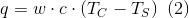w = blood mass flow rate [kg/s]

c = heat capacity of blood [J/(kg·K)]

TC  = body core temperature [K]

Equating equation (1) and (2) yields toDividing by surface area A: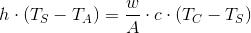where p = perfusion rate [kg/(s·)].

Solving for TC:According to the US patent US 6299347 B1 h/(p∙c) can be expressed as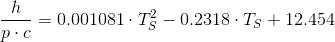which is an approximation of h/(p·c) with change in skin temperature for afebrile and febrile ranges.

Substituting h/(p·c) in equation (3) we finally get the body core temperature in °F by the forehead and ambient temperature in °F: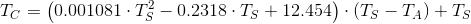6. Respiration rate sensor

Basically the sensor consists of a disposable respirator- half-mask, a 3-D printed connection nipple, a piece of silicone hose and the pressure sensor MPXV4006GP. The advantage of this setup is that the repiration rate also can be measured during activities like running and the exhaled gases could be analyzed by a gas sensor at the same time.

The connection nipple were printed on an industrial grade 3-D-printer:

Connection nipple and silicone hose assembled on mask:

The gauge pressure rises only approx. 10 mm H2O when breathing out, so the output voltage of the pressure sensor must be amplified. This is done by a non-inverting amplifier:Vout is given by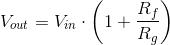I chose a gain of 11, which works just fine. The circuit was then built on a small perf board for testing:

Example code:

```unsigned long resp_delta;
unsigned int resp_counter = 0;
byte led = 13;
byte resp_sense = A0;
void setup() {
Serial.begin(9600);
pinMode(led, OUTPUT);
}
void loop() {
boolean resp_state = 0;
resp_state = 1;
digitalWrite(led, HIGH);
delay(300);
}
}
if(resp_state == 1) {
resp_counter ++;
if(resp_counter == 1) {
resp_delta = millis();
}
if(resp_counter == 11) {
resp_delta = millis();
unsigned int respiratory_rate = 600000 / (resp_delta - resp_delta);
Serial.print(“Respiratory rate: “);
Serial.print(respiratory_rate);
Serial.println(” bpm”);
resp_counter = 0;
}
resp_state = 0;
}
digitalWrite(led, LOW);
}
```

7. Blood type calculator

Do you know your blood type and Rh factor? I always forget mine. Somewhere I should have a vaccination record, but where? I forget as well, where I put it.
Is there a way to evaluate my blood type and Rh factor without a blood test? If you know the blood types and Rh factors of your parents, you can at least limit the blood type and Rh factor you may have, using following algorithm and you can compute the highest probability, because blood types and Rh factors are not equally distributed.

I started with a ABO blood type and Rh factor look-up table, transformed the tables into 2-dimensional char arrays and by studying the blood type distribution by country and the weighted mean, not only all possible blood types and Rh factors but also the ones with the maximum probability could be easily computed.

```// your input:
String father_bloodtype = “AB”;
String mother_bloodtype = “AB”;
String father_RHtype = “-/-”;
String mother_RHtype = “-/-”;
void setup() {
Serial.begin(9600);
char* bloodtypes[] = {“A”, “B”, “AB”, “O”};
char* RHtypes[] = {"+/+", “+/-”, “-/-”};
char* your_bloodtype =
{
{“A, B”,  “A, B, AB, O”,  “A, B, AB”, “A, O”},
{“A, B, AB, O”,  “B, O”,  “A, B, AB”, “B, O”},
{“A, B, AB”,  “A, B, AB”,  “A, B, AB”, “A, B”},
{“A, O”,  “B, O”,  “A, B”, “O”}
};
char* your_bloodtype_prob =
{
{“A”,  “O”,  “A”, “O”},
{“O”,  “O”,  “A”, “O”},
{“A”,  “A”,  “A”, “A”},
{“O”,  “O”,  “A”, “O”}
};
char* your_RHtype =
{
{"+/+",  “+/+, -/-”,  “+/-”},
{"+/+, -/-",  “+/+, +/-, -/-”,  “+/-, -/-”},
{"+/-",  “+/-, -/-”,  “-/-”}
};
char* your_RHtype_prob =
{
{"+",  “+”,  “+”},
{"+",  “+”,  “+”},
{"+",  “+”,  “-”}
};
byte father_row;
byte mother_col;
for(byte i = 0; i < 4; i ++) {
if(father_bloodtype.compareTo(bloodtypes[i]) == 0) father_row = i;
if(mother_bloodtype.compareTo(bloodtypes[i]) == 0) mother_col = i;
}
Serial.print("Possible blood types: ");
Serial.println(your_bloodtype[father_row][mother_col]);
Serial.print("Blood type with highest probability: ");
Serial.println(your_bloodtype_prob[father_row][mother_col]);
for(byte i = 0; i < 3; i ++) {
if(father_RHtype.compareTo(RHtypes[i]) == 0) father_row = i;
if(mother_RHtype.compareTo(RHtypes[i]) == 0) mother_col = i;
}
Serial.print("Possible Rh factors: ");
Serial.println(your_RHtype[father_row][mother_col]);
Serial.print("Rh factor with highest probability: ");
Serial.println(your_RHtype_prob[father_row][mother_col]);
}
void loop() {
}
```

8. Shield design

A lot of goodies have been added on the new shield compared to the previous design:

• Pressure sensor MPXV4006GP
• Two color 128x64 OLED
• LED to display respiration rate
• SMD piezo buzzer
• SMD audio jack to connect heart rate sensor

Bare PCB, 10 pieces 50 RMB without express: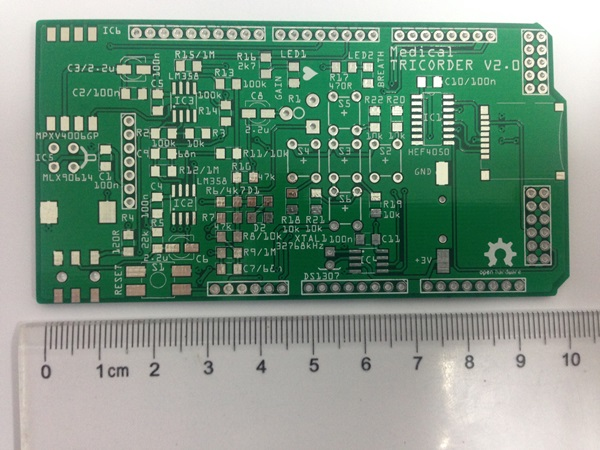Populated PCB without OLED:

PCB with OLED:

9. Future work

The next iteration of the medical tricorder will contain a bacteria sensor based on a photometer. Some basic work regarding exponential bacteria growth modeling has been done.

Let donate the increase in cells numbers ΔN per time interval Δt, then this ratio is proportional to the actual number of cells N. If for example a population of 10000 cells produces 1000 new cells per hour, a 3 times bigger population of the same microorganism will produce 3000 new cells per hour.Written as a differential:Using a proportionality factor μ<0, which is called specific growth rate, yields to the first-order ordinary differential equation:Separating the variables yieldsIntegrating both sides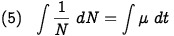Determining the integration constant c using the initial condition t=0: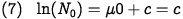Substituting c in equation (6):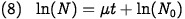Solving for N:Let donate the generation time tg, where exactly N=2N0, the equation (8) yieldsSubstituting μ in equation (9):Using following selected bacterium generation times a simple bacteria growth simulator was written

 Bacterium Generation time [h] Beneckea natriegens 0.16 Escherichia coli 0.35 Bacillus subtilis 0.43 Staphylococcus aureus 0.47 Clostridium botulinum 0.58
```void setup() {
Serial.begin(9600);
}
void loop() {
const unsigned int N_0 = 100; // population at t = 0
char* bacteria[] = {“Beneckea natriegens”, “Escherichia coli”, “Bacillus subtilis”,
“Staphylococcus aureus”, “Clostridium botulinum”}; // bacteria
float t_g[] = {0.16, 0.35, 0.43, 0.47, 0.58}; // generation time in hours
for(byte i = 0; i < 5; i++) {
Serial.print(bacteria[i]);
Serial.println(":");
for(byte j = 0; j < 5; j++) {
unsigned long N = N_0 * long(pow(2.0,float(j)/t_g[i]));
Serial.print(j);
Serial.print(" h: “);
Serial.print(N);
if(j < 4) Serial.print(”, “);
else Serial.println(”");
delay(1000);
}
Serial.println("");
}
}
```

10. References

Data logging

Data is logged via serial? Future iterations may have a sd card slot to gather data.

Another cool sensors would be alcohol and carbon monoxide sensors.

1 Like

The shield has a micro SD

The shield has a micro SD card slot already and data can be logged.

I was browsing at low

I was browsing at low luminosity and didn’t see sd cardThe output file is xml, a database or a flat csv?

**Interesting.

However, I’d**
Interesting.

However, I’d check the values of the lowest and highest floating point values for the system. Forgive me, but I’m working off theory I learned in the 70s and early 80s because I was a geek and couldn’t find a girlfriend during most of that period.

Basically you get more data loss from addition than multiplication with floating point numbers.

The floats used in computing usually have enough range for the numbers you’ve shown for multiplication. However, because there is a separate mantissa and exponent (something like 1.45 * (2 to the 63rd) is an example), adding a very small number to a very large number can loss the small number entirely. Therefore if you’re using a small enough float (32 bits) and your range of numbers is high, it is more accurate to add numbers in the order of increasing size.

64 bit floating point is usually enough to prevent most of the data loss due to lack of precision. It should be rare that you have to use logarithms to multiply numbers to keep precision unless you’ve taught your device to run a virtual slide rule.Hi Silux, I am using a .txt

Hi Silux, I am using a .txt file at the moment.

Hi,

I was not talking about precision. I was talking about floating-point underflow which could occur when multiplying a lot of probabilities. In data mining you often have to deal with thousands or even millions of probabilities and using log probabilities is a commonly used technique. Letand using the natural logarithmWe can rewrite the inequality asand see immediately that we just need to add the exponents on both sides of the inequality as all bases of the four numbers are forced to e (Euler's number):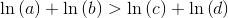Bearing in mind, that for example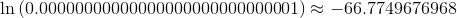it should be clear what advantage it has to use log probabilities.